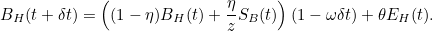# The effect of recent criminal activity

We want to model the effect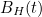of recent criminal activity on house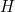. First of all, let’s assume that every time a houseis burgled,increases by an amountThis increase isn’t going to last forever though: it’s going to diminish over time. Write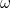for the time scale over which repeat burglaries are likely to occur in a neighbourhood.

Now write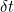for the length of a time step. So what we’re after is a formula for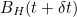in terms of. The formula used by Bertozzi and her colleagues is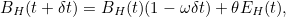where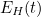is the number of burglaries that happened at houseat the time. You can see that each of these burglaries contributes an amount ofBurglaries that happened before that time are accounted for by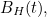but the amount by which they contribute is determined by the relationship ofand the length of the time step. The larger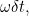the smaller the contribution of(we assume that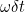is less than).

This formula only reflects how recent burglaries at houseaffect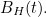But we also need to take account of the effect of recent burglaries at neighbouring houses. To do this, replacein the equation above by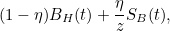where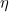is a number between 0 and 1 that measures how significant the effect of crimes in adjacent houses is. The parameter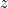is the number of houses that directly neighbour(so in a square lattice that number is four).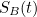is the sum of the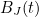for all the houses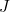adjacent to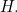The formula now becomes